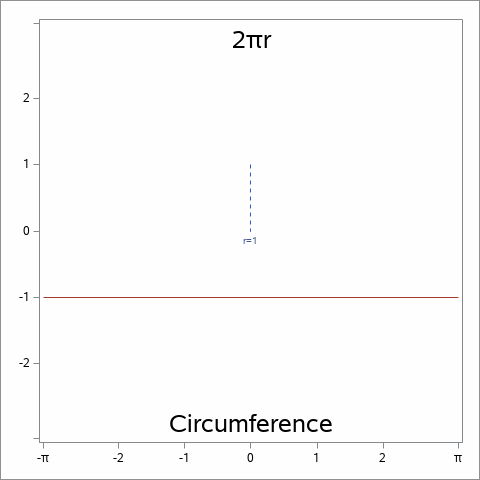## Fun w/SAS ODS Graphics: Fold line of length 2*PI*r into a circleFold line of length 2*PI*r into a circle

Inspired by a @Rick_SAS  blog post, here's a last-minute PI Day chart (hey, it's still PI Day in Hawaii!).

``````*==> Fun w/SAS ODS Graphics: Fold line of length 2*PI*R into a circle;

data circle;                                          * Calc x/y points for circle of N points;
retain xR yR xRO 0 yRO 1 tR "r=1";                    * Used to draw unit circle radius;
seq=0; xo=-constant("pi"); yo=-1; x=-xo; y=yo; output; x=.; * Starting line segment of length 2*PI;
N=40;                                                 * Number of line segments in circle (40+ is good, multiples of 4);
d=constant("pi");                                     * Loop for points on right side of circle;
do a=-2*constant("pi")/4 to 2*constant("pi")/4 by 2*constant("pi")/N;
x=cos(a);                                           * Assume unit circle with radius=1;
y=sin(a);
xo=cos(lag(a));                                     * Prior x/y point;
yo=sin(lag(a));
if xo^=. then do;                                   * Output circle line segments;
seq+1;
d=d-2*constant("pi")/N;                           * "Unused" line segment (drawn perpindicular to last points on circle);
m=-1/(y/x);                                       * Slope of tangent to current point on circle;
if m=. then do;                                   * Slope=infinity (x=0)?;
xT=x;                                           * Yes, calculate x/y endpoint of "unused" line;
yT=y+d;
end;
else do;                                          * No, calculate x/y endpoint of "unused" line;
xT=x+sign(m)*d/sqrt(1+m**2);                    * Slope determines x direction;
yT=y+abs(m)*d/sqrt(1+m**2);                     * y direction always positive;
end;
output;
x=-x;                                             * Negate x values for left side of circle;
xo=-xo;
xT=-xT;
output;
end;
end;

proc sql;                                             * Determine frames needed for GIF;
create table framenums as select distinct seq as frame from circle;

create table frames as                                /* Create frames with needed points */
select frame, seq, x, y, xo, yo, xR, yR, tR, xRO, yRO,
case when f.frame=c.seq then xT end as xT, case when f.frame=c.seq then yT end as yT
from framenums f, circle c where f.frame>=c.seq and (f.frame=0 or c.seq>0) order by 1, 2;

ods _all_ close;                                      * Animated GIF setup;
options papersize=('5 in', '5 in') printerpath=gif animation=start
nodate nonumber animloop=YES NOANIMOVERLAY animduration=.5;
ods printer file='/folders/myfolders/piDayV2.gif';
ods graphics / width=5in height=5in imagefmt=GIF;

%macro genCircle(start=, stop=, dur=);
options nobyline animduration=&dur;                   * Create plots;
proc sgplot data=frames aspect=1 noautolegend;
by frame;
where &start<=frame<=&stop;
vector x=xR y=yR / xorigin=xRO yorigin=yRO            /* Radius */
vector x=x y=y / xorigin=xo yorigin=yo noarrowheads;  /* Segments of circles */
vector x=x y=y / xorigin=xT yorigin=yT noarrowheads;  /* Remaining "unused" line segment */
xaxis display=(nolabel) min=-3.25 max=3.25 offsetmin=.01 offsetmax=.01
values=(-3.14 -2 -1 0 1 2 3.14) valuesdisplay=("-(*ESC*){Unicode pi}" "-2" "-1" "0" "1" "2" "(*ESC*){Unicode pi}");
yaxis display=(nolabel) min=-3.25 max=3.25 offsetmin=.01 offsetmax=.01
values=(-3.14 -2 -1 0 1 2 3.14) valuesdisplay=(" " "-2" "-1" "0" "1" "2" " ");
inset "2(*ESC*){Unicode pi}r" / position=top textattrs=(size=18pt);
inset "Circumference" / position=bottom textattrs=(size=18pt);
run;
%mend;
%genCircle(start=0, stop=0, dur=.5);                  * Start frame (.5 seconds);
%genCircle(start=0, stop=20, dur=.15);                * Draw circle (.15 seconds/frame);
%genCircle(start=20,stop=20, dur=.5);                 * Final frame (.5 seconds);

options printerpath=gif animation=stop;               * Animated GIF wrapup;
ods printer close;``````

2 REPLIES 2

## Re: Fun w/SAS ODS Graphics: Fold line of length 2*PI*r into a circle

Using an invisible REFLINE to get custom labels is a neat trick. You can achieve the same result by using the

VALUES and VALUESDISPLAY option on the XAXIS and YAXIS statements. No need for invisible lines.

## Re: Fun w/SAS ODS Graphics: Fold line of length 2*PI*r into a circle

Right, you are, Rick! Modified above code/image (tossed in a radius line and titles, too).

Discussion stats
• 2 replies
• 731 views
• 9 likes
• 2 in conversation